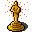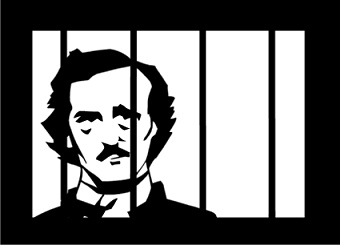Writers' Block Poetry Night2011 Slam Season# Rae Wallace - 2011 Writers' Block Women of the World Grand Slam Champ

All our reps to national slams have expenses. Support Rae as she brings it home in March.Below, see how as the pool of candidates grew from week to week, all vying to be the representative at the 2011 Women of the World Poetry SlamWOWps 2011 PoolSept. 22, 2010 Slam (3 minute & 1 minute rounds)
1st  Gina Blaurock - 3 points (27.6 + 28.1 = 55.7)
2nd  Paula Lambert - 2 points (28 + 27.2 = 55.2)
3rd  (tie with Vernell) Rachel Wiley - 1 point (27.9 - .5 TP + 27.7 = 55.1)
3rd  (tie with Rachel) Vernell Bristow - 1 point (26.1 + 29 = 55.1)
--------------------------------------------------------------
5th - Louise Robertson (26.8 + 28.1 = 54.9)
6th - Rae Wallace (26.8 + 27.1 = 53.9)
7th - Claudia Wilson (24.5 + 27.6 = 52.1)
8th - Katrina K. (24.5 + 26.9 = 51.4)

Sept. 29, 2010 Slam (3 minute & 2 minute rounds)
1st  Vernell Bristow - 3 points (29.4 + 29.3 = 58.7)
2nd  Louise Robertson - 2 points (27.2 + 28.9 = 56.1)
3rd  Gina Blaurock - 1 point (27.3 + 28.1 = 55.4)
--------------------------------------------------------------
4th - Paula Lambert (27.5 + 26.1 = 53.7)
5th - Rae Wallace (26.4 + 26.9 = 53.3)
6th - Katrina K. (25.6 + 26.6 = 52.2)

October 13, 2010 Slam (3 minute & 3 minute rounds)

1st  Rose Smith - 3 points (30 + 27.1 = 57.1)
2nd  Louise Robertson - 2 points (27.7 + 28.9 = 56.6)
3rd  Gina Blaurock - 1 point (27.0 + 29.4 = 56.4)
--------------------------------------------------------------
4th - Paula Lambert (26.7 + 28.3 = 55.0)
5th - Rachel Wiley (27.3 + 27.5 = 54.8)
6th - Missy Conley (28.4 + 25.5 = 53.9)
7th - Claudia Wilson (26 + 26.3 = 52.3)
8th - Vernell Bristow (24.9 + 26.2 = 51.1)
9th - Noa (22.8 + 25.6 = 48.4)

October 20, 2010 Slam (2 minute & 1 minute rounds)
1st  Vernell Bristow - 3 points (26.3 + 25.1 = 51.4)
2nd  Rae Wallace - 2 points (25.7 + 25.3 = 51.0)
3rd  Rachel Wiley - 1 point (24.8 + 25.9 = 50.7)
--------------------------------------------------------------
4th - Katrina K. (24.3 + 26.0 = 50.3)
5th - Paula Lambert (25.7 + 24.4 = 50.1)
6th - Gina Blaurock (24.1 + 25.6 = 49.7)
7th - Louise Robertson (23.4 + 25.3 = 48.7)
8th - Missy (23.2 + 24.3 = 24.5)

November 17, 2010 Slam (2 minute & 3 minute rounds)
1st  Rose Smith - 3 points (28.0 + 27.9 = 55.9)
2nd  Rachel Wiley - 2 points (26.2 + 30.0 - 1.0 TP = 55.2)
3rd  Louise Robertson - 1 point (26.9 + 27.7 = 54.6)
--------------------------------------------------------------
4th - Missy (26.1 + 28.4 = 54.5)
5th - Gina Blaurock (26.5 + 27.4 = 53.9)
6th - Katrina K. (26.2 + 27.1 = 53.3)
7th - Rae Wallace (26.6 + 26.3 = 52.9)
8th - Lois (25.2 - .5 TP + 24.5 - 1.5 TP = 47.7)

Total Points Awarded
The top 8 poets listed below will be in the Grand Slam
Wednesday, December 15, 2010

1) Vernell Bristow - 7 pt.
2) Rose Smith - 6 pts.
3) Gina Blaurock - 5 pt.
3) Louise Robertson - 5 pt.
5) Rachel Wiley - 4 pt.
6) Rae Wallace - 2 pt.
6) Paula Lambert - 2 pt.

* Bracketed poets have not yet met the 2 slam requirement.

December 15, 2010 GRAND SLAM Results
1st and WOWps rep - Rae Wallace (25.9 + 25.7 + 27.9 = 79.5)
2nd - Vernell Bristow (25.2 +27.7 + 26.3 = 79.2)
3rd - Rose Smith (27.2 + 25.1 + 26.4 = 78.7 )
4th - Rachel Wiley (24.2 + 27.7 + 26 = 77.9)
5th - Gina Blaurock (24.8 + 25.7 + 26.7 = 76.8)
6th - Paula Lambert (23.3 + 25.5 + 27.5 - .5 tp = 75.8)
7th - Louise Robertson ( 23.7 + 24.6 + 24.3 = 72.6)